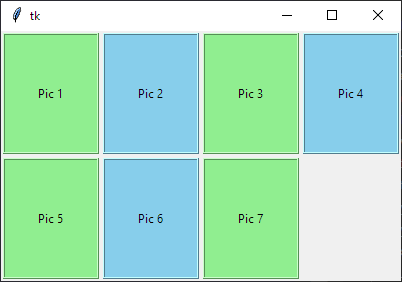# How to flex-wrap items in tkinter

Hi!
Could you help me please solve this problem? I have a list of images (each image is unique) and I want to display all images in tkinter window. The problem is if I have more than 5 images, the 6th image is out of window (if I want to see this image I have to resize window → not very optional).
In CSS is this awesome idea of flex-box and wrap, that looks like this:

``````.container {
display: flex;
flex-wrap: wrap;
}

.element {
flex: 0 0 200px; /* Adjust the width of each element as needed */
margin: 10px;
}
``````

I need to apply same method in tkinter.
If you know what would solve my problem I would be really gladhere is my code:

``````import tkinter as tk

class MyApplication:
def __init__(self):
self.window = tk.Tk()

# Create a list of image paths
image_paths = [
]

# Create a list of PhotoImage objects
self.images = [tk.PhotoImage(file=path).subsample(4, 4) for path in image_paths]

num_images = len(image_paths)

# Create a container frame to hold the frames with image-button pairs
container = tk.Frame(self.window)
container.pack(fill=tk.BOTH, expand=True)

for i in range(num_images):
# Create a Frame to hold the picture and button
frame = tk.Frame(container)

image = self.images[i]

# Create a Label to hold the picture
image_label = tk.Label(frame, image=image)
image_label.pack(side=tk.TOP)  # Place the picture at the top of the frame

button = tk.Button(frame, text=f"Button {i+1}", command=lambda id=i+1: self.button_click(id))
button.pack(side=tk.BOTTOM)  # Place the button at the bottom of the frame

def button_click(self, button_id):
print("Button clicked:", button_id)

def run(self):
self.window.mainloop()

# Create an instance of the application
app = MyApplication()
app.run()
``````

I would use tkinters grid instead of pack. You can place x amount of images in one row then drop to another and start again.

Example:

``````import tkinter as tk

class App:
def __init__(self, parent):
parent.columnconfigure(0, weight=1)
parent.rowconfigure(0, weight=1)

numbers = [num for num in range(7)]

container = tk.Frame(parent)
container.grid(column=0, row=0, sticky='news')
for i in range(4):
container.grid_columnconfigure(i, weight=3, uniform='pics')

col = 0
row = 0

for number in numbers:
bg = 'lightgreen' if number % 2 == 0 else 'skyblue'
pic = tk.Label(container, text=f'Pic {number+1}', bg=bg, relief='ridge')
if col == 3:
col = 0
row += 1
else:
col += 1
container.grid_rowconfigure(row, weight=3, uniform='rows')

root = tk.Tk()
root.geometry('400x250+300+300')
App(root)
root.mainloop()
``````